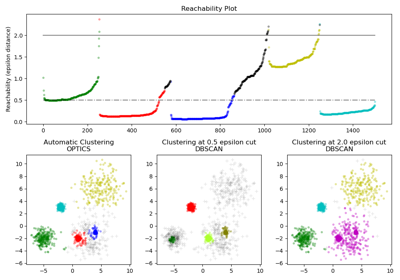# sklearn.cluster.cluster_optics_dbscan¶

sklearn.cluster.cluster_optics_dbscan(*, reachability, core_distances, ordering, eps)[source]

Performs DBSCAN extraction for an arbitrary epsilon.

Extracting the clusters runs in linear time. Note that this results in labels_ which are close to a DBSCAN with similar settings and eps, only if eps is close to max_eps.

Parameters
reachabilityarray, shape (n_samples,)

Reachability distances calculated by OPTICS (reachability_)

core_distancesarray, shape (n_samples,)

Distances at which points become core (core_distances_)

orderingarray, shape (n_samples,)

OPTICS ordered point indices (ordering_)

epsfloat

DBSCAN eps parameter. Must be set to < max_eps. Results will be close to DBSCAN algorithm if eps and max_eps are close to one another.

Returns
labels_array, shape (n_samples,)

The estimated labels.

## Examples using sklearn.cluster.cluster_optics_dbscan¶